# How to Calculate the Area, Radius and Height of a Cylinder | Nickzom Calculator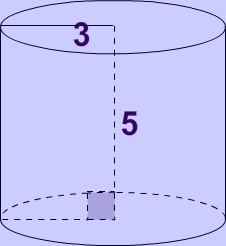The image above represents a cylinder.
To compute the area of a cylinder requires two essential parameters which are the radius and height of the cylinder.

The formula for computing the area of a cylinder is:

A = 2πrh + 2πr2

Where:
A = Area of a cylinder
r = radius of the cylinder
h = height of the cylinder

Let’s solve an example
Find the area of a cylinder with a radius of 3 cm and a height of 5 cm.

This implies that:
r = radius of the cylinder = 3
h = height of the cylinder = 5

A = 2πrh + 2πr2
A = 2(3.142) x 3 x 5 + 2(3.142) x 32
A = 150.82

Therefore, the area of the cylinder is 150.82 cm2.

Calculating the Height of a cylinder when Area and Radius is Given

The formula is h =  A/ 2πr – r

Where;
A = Area of a cylinder
r =  radius of the cylinder
h = height of the cylinder

Let’s solve an example:
Find the height of a cylinder with an area of 600 cm² and a radius of 5 cm

This implies that;
A = Area of the cylinder = 600 cm²
r  = radius of the cylinder = 5 cm

h = A / 2πr – r
h = 600 / 2(3.142)(5) – 5
h = 600 / 31.42 – 5
h = 19.10 – 5
h = 14.10

Therefore, the height of the cylinder is 14.10 cm.

Nickzom Calculator – The Calculator Encyclopedia is capable of calculating the area of a cylinder.

To get the answer and workings of the area of a cylinder using the Nickzom Calculator – The Calculator Encyclopedia. First, you need to obtain the app.

You can get this app via any of these means:

To get access to the professional version via web, you need to register and subscribe for NGN 1,500 per annum to have utter access to all functionalities.
You can also try the demo version via https://www.nickzom.org/calculator

Once, you have obtained the calculator encyclopedia app, proceed to the Calculator Map, then click on Mensuration under the Mathematics section.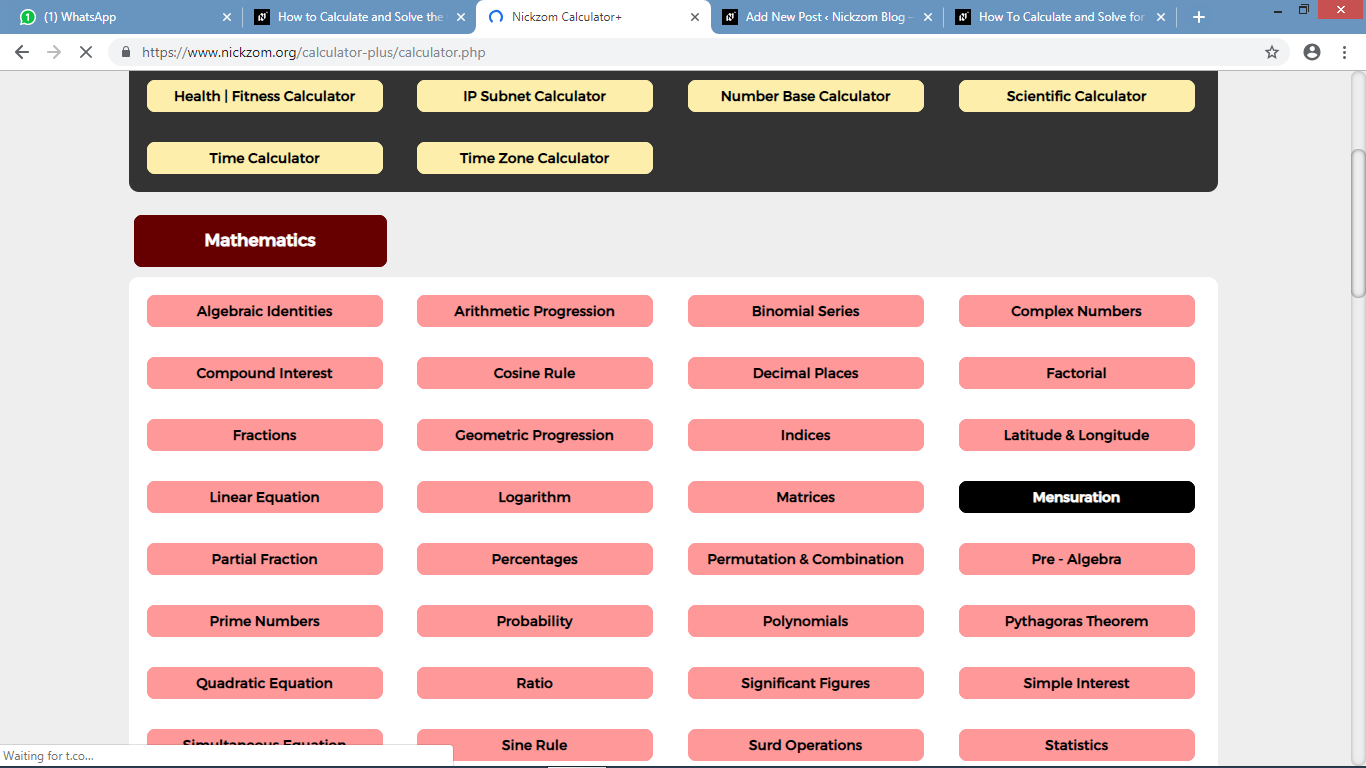Now, click on Area of a Cylinder under Mensuration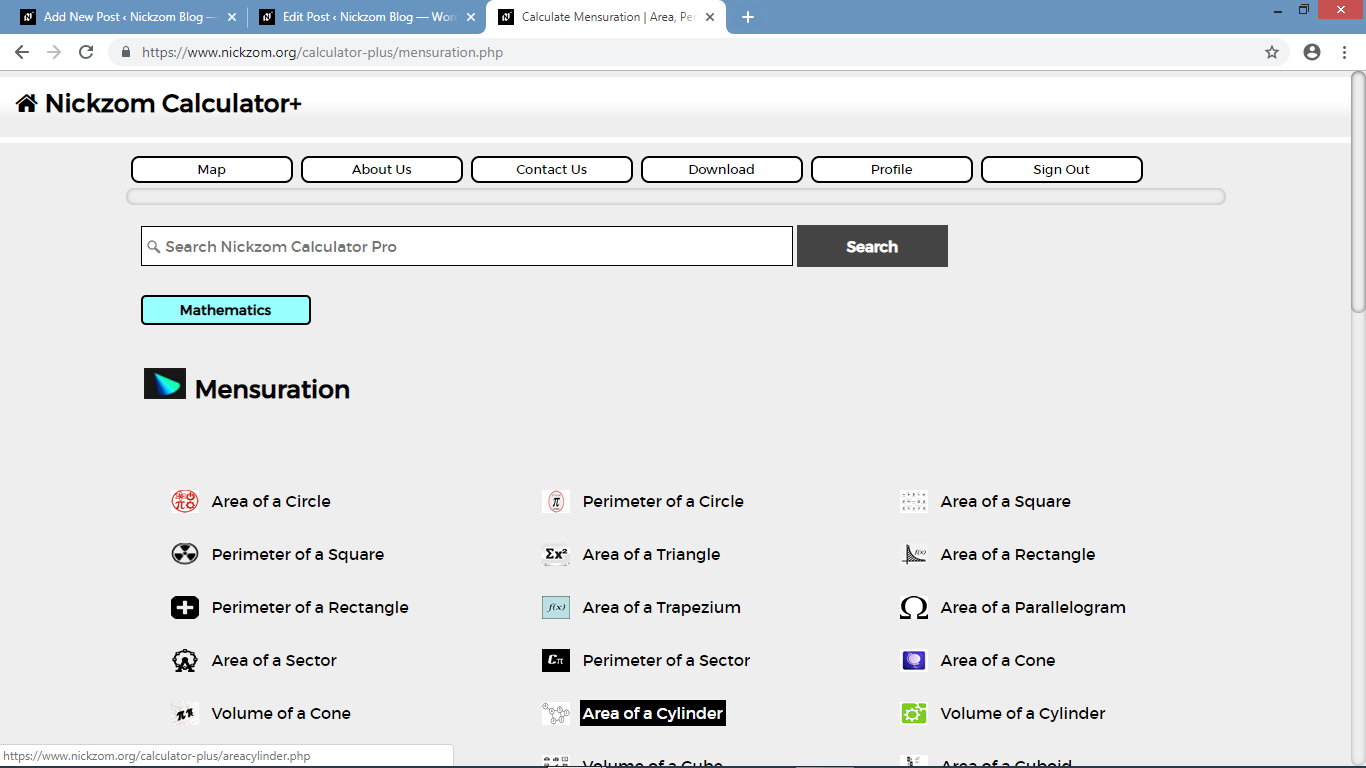The screenshot below displays the page or activity to enter your values, to get the answer for the area of a cylinder according to the respective parameters which are the radius and height of the cylinder.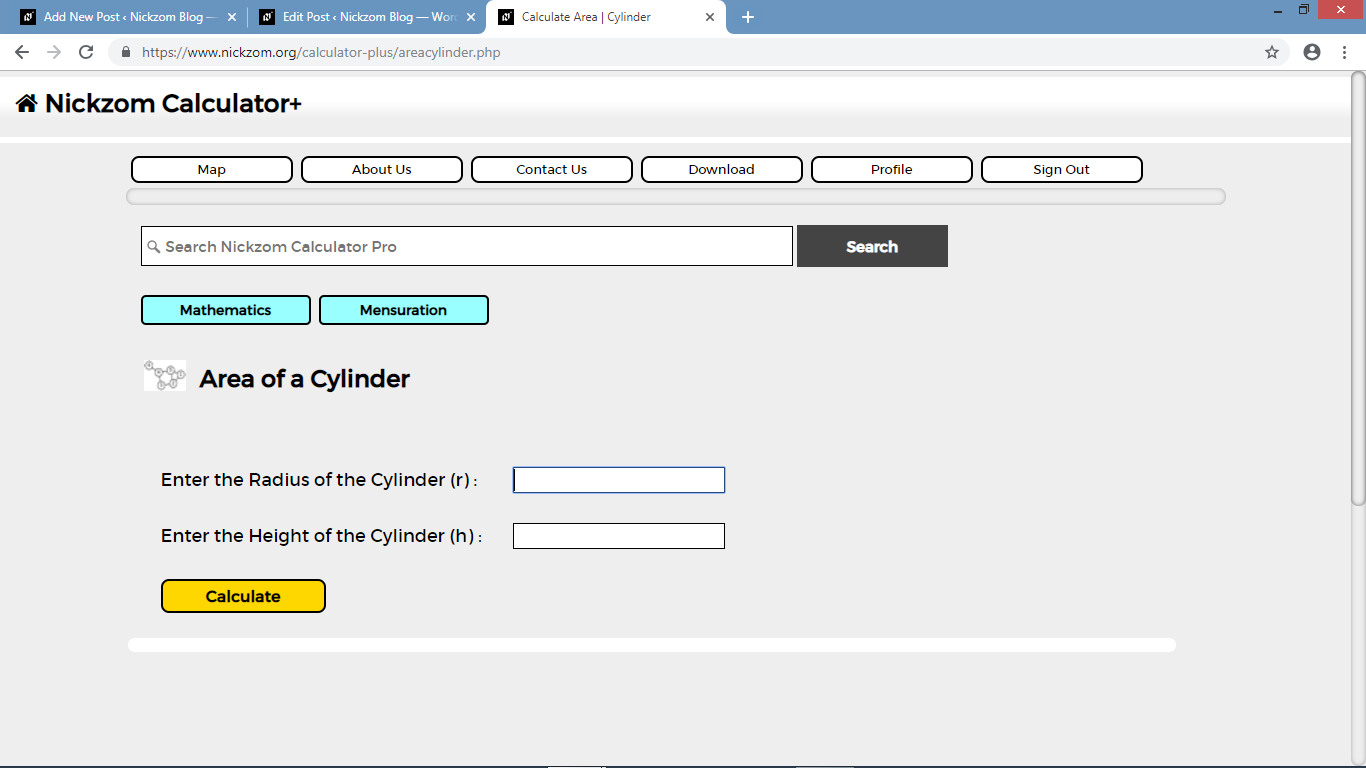Now, enter the values appropriately and accordingly for the parameters as required by the example above where the radius of the cylinder is 3 cm and the height of the cylinder is 5 cm.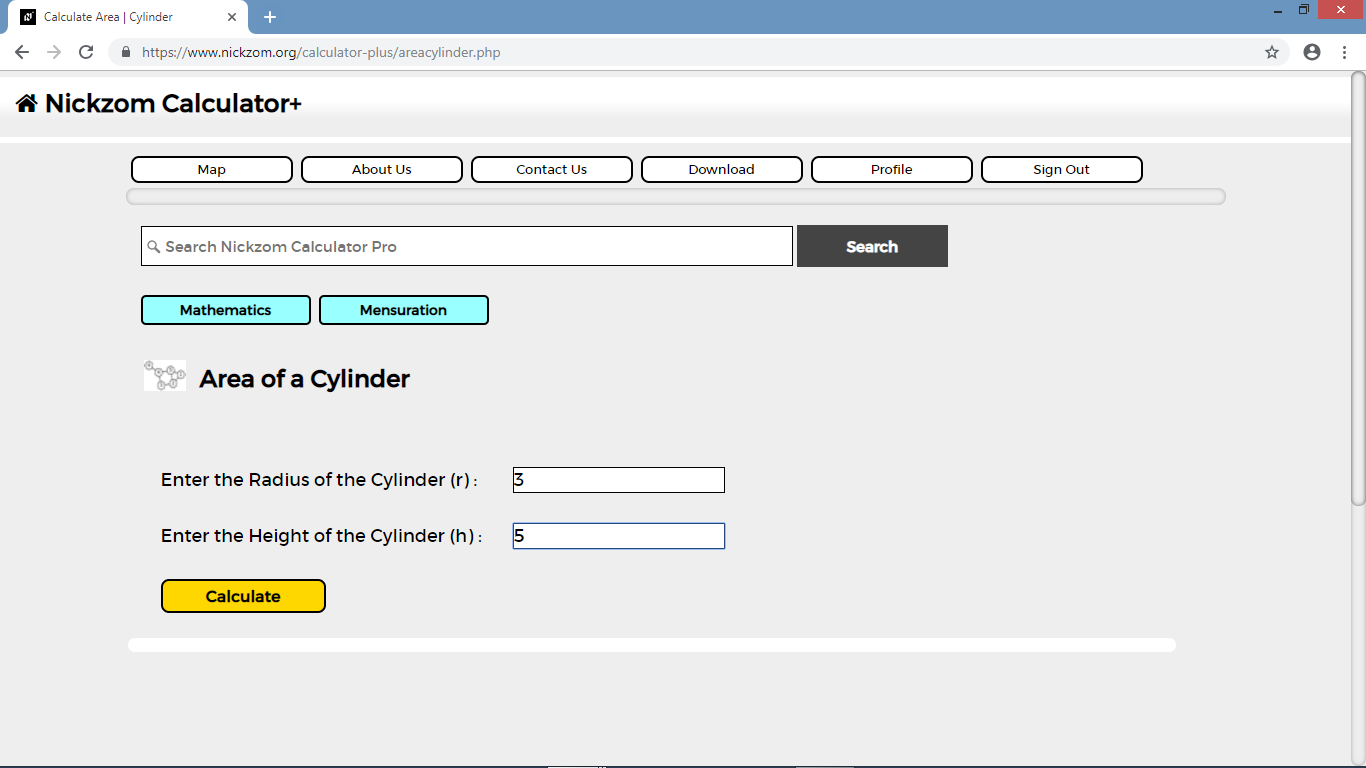Finally, click on the Calculate button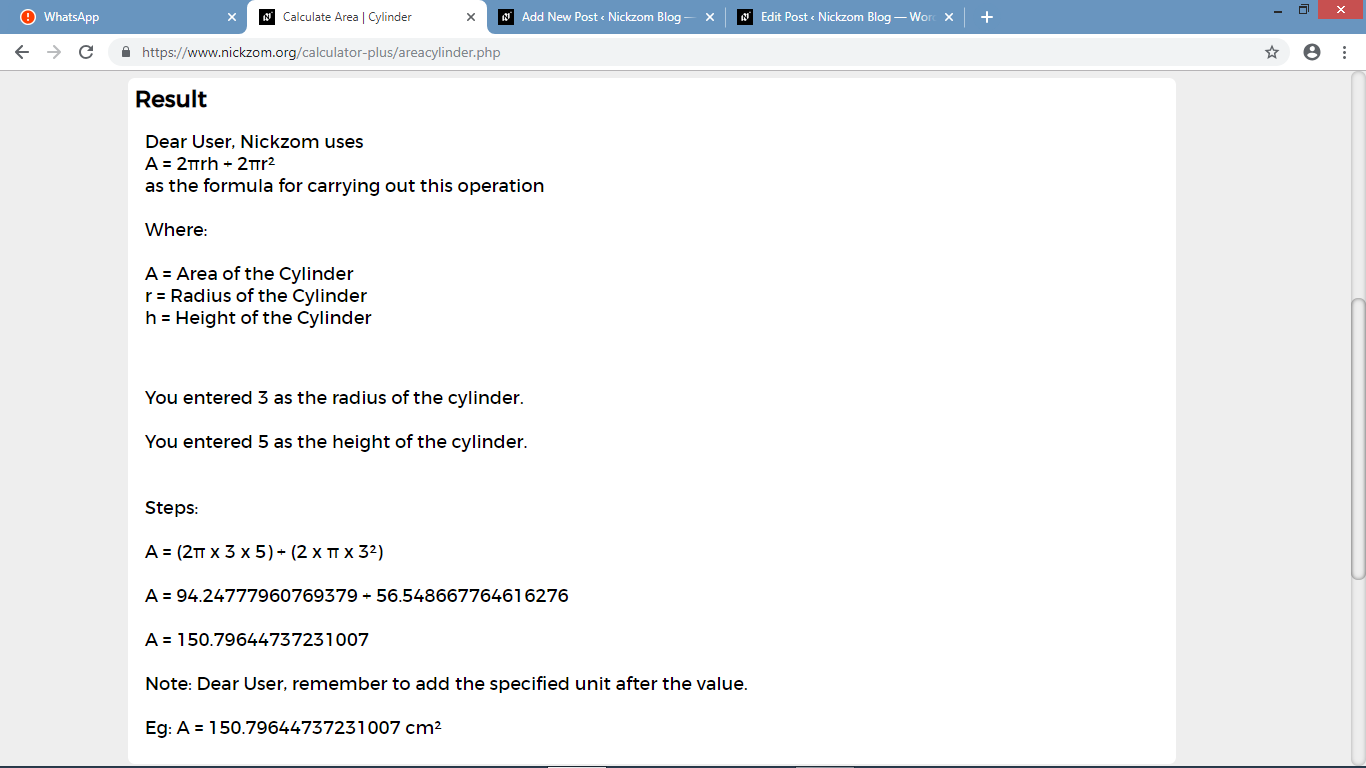As you can see from the screenshot above, Nickzom Calculator – The Calculator Encyclopedia solves for the area of a cylinder and presents the formula, workings and steps too.